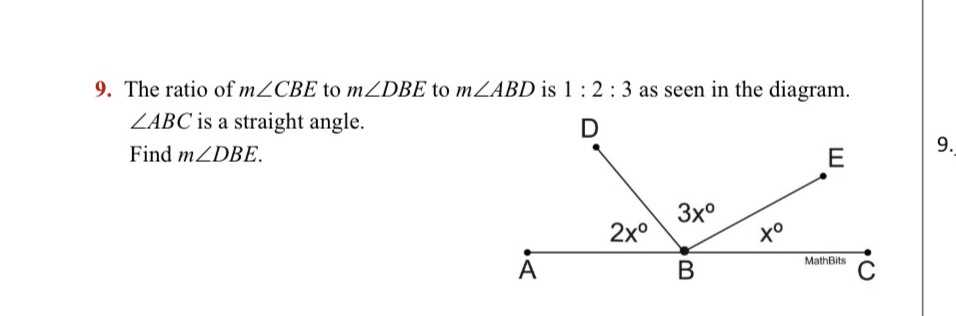### ¿Todavía tienes preguntas de matemáticas?

Pregunte a nuestros tutores expertos
Algebra
Pregunta9. The ratio of $$m \angle C B E$$ to $$m \angle D B E$$ to $$m \angle A B D$$ is $$1 : 2 : 3$$ as seen in the diagram.

$$\angle A B C$$ is a straight angle.. Find $$m \angle D B E$$